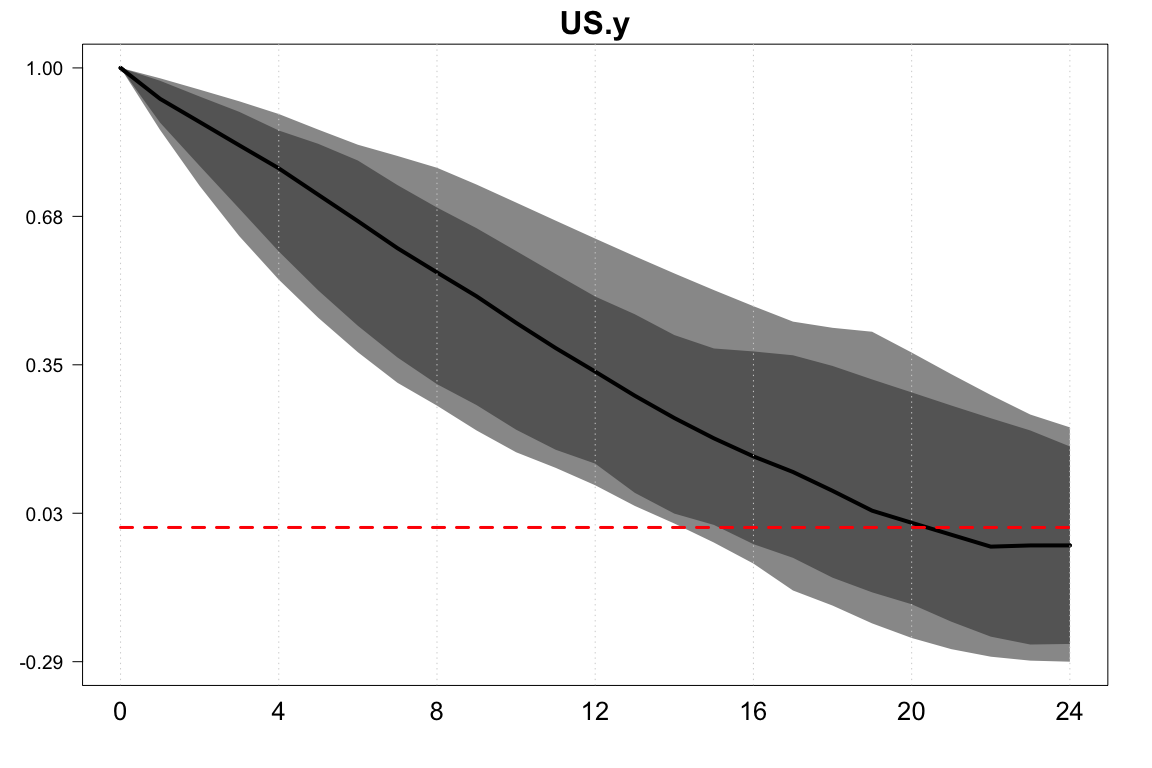# BGVAR: Bayesian Global Vector Autoregression

#### 02 Mai 2022

Abstract

This document describes the BGVAR library to estimate Bayesian Global vector autoregressions (GVAR) with different prior specifications and stochastic volatility. The library offers a fully fledged toolkit to conduct impulse response functions, forecast error variance and historical error variance decompositions. To identify structural shocks in a given country model or joint regional shocks, the library offers simple Cholesky decompositions, generalized impulse response functions and zero and sign restrictions – the latter of which can also be put on the cross-section. We also allow for different structures of the GVAR like including different weights for different variables or setting up additional country models that determine global variables such as oil prices. Last, we provide functions to conduct and evaluate out-of-sample forecasts as well as conditional forecasts that allow for the setting of a future path for a particular variable of interest. The toolbox requires R>=3.5.

# Introduction

This vignette describes the BGVAR package that allows for the estimation of Bayesian global vector autoregressions (GVARs). The focus of the vignette is to provide a range of examples that demonstrate the full functionality of the library. It is accompanied by a more technical description of the GVAR framework. Here, it suffices to briefly summarize the main idea of a GVAR, which is a large system of equations designed to analyze or control for interactions across units. Most often, these units refer to countries and the interactions between them arise through economic and financial interdependencies. Also in this document, the examples we provide contain cross-country data. In principle, however, the GVAR framework can be applied to other units, such as regions, firms, etc. The following examples show how the GVAR can be used to either estimate spillover effects from one country to another, or alternatively, to look at the effects of a domestic shock controlling for global factors.

In a nutshell, the GVAR consists of two stages. In the first, $$N$$ vector autoregressive (VAR) models are estimated, one per unit. Each equation in a unit model is augmented with foreign variables, that control for global factors and link the unit-specific models later. Typically, these foreign variables are constructed using exogenous, bilateral weights, stored in an $$N \times N$$ weight matrix. The classical framework of Pesaran, Schuermann, and Weiner (2004) and Dees et al. (2007) proposes estimating these country models in vector error correction form, while in this package we take a Bayesian stance and estimation is carried out using VARs. The user can transform the data prior estimation into stationary form or estimate the model in levels. The BGVAR package also allows us to include a trend to get trend-stationary data. In the second step, the single country models are combined using the assumption that single models are linked via the exogenous weights, to yield a global representation of the model. This representation of the model is then used to carry out impulse response analysis and forecasting.

This vignette consists of four blocks: getting started and data handling, estimation, structural analysis and forecasting. In the next part, we discuss which data formats the bgvar library can handle. We then proceed by showing examples of how to estimate a model using different Bayesian shrinkage priors – for references see Crespo Cuaresma, Feldkircher, and Huber (2016) and Feldkircher and Huber (2016). We also discuss how to run diagnostic and convergence checks and examine the main properties of the model. In the third section, we turn to structural analysis, either using recursive (Cholesky) identification or sign restrictions. We will also discuss structural and generalized forecast error variance decompositions and historical decompositions. In the last section, we show how to compute unconditional and conditional forecasts with the package.

# Getting Started

We start by installing the package from CRAN and attaching it with

oldpar <- par(no.readonly=TRUE)
set.seed(123)
library(BGVAR)

To ensure reproducibility of the examples that follow, we have set a particular seed (for Rs random number generator). As every R library, the BGVAR package provides built-in help files which can be accessed by typing ? followed by the function / command of interest. It also comes along with four example data sets, two of them correspond to data the quarterly data set used in Feldkircher and Huber (2016) (eerData, eerDataspf), one is on monthly frequency (monthlyData). For convenience we also include the data that come along with the Matlab GVAR toolbox of Smith, L.V. and Galesi, A. (2014), pesaranData. We include the 2019 vintage (Mohaddes and Raissi 2020).

We start illustrating the functionality of the BGVAR package by using the eerData data set from Feldkircher and Huber (2016). It contains 76 quarterly observations for 43 countries over the period from 1995Q1 to 2013Q4. The euro area (EA) is included as a regional aggregate.

We can load the data by typing

data(eerData)

This loads two objects: eerData, which is a list object of length $$N$$ (i.e., the number of countries) and W.trade0012, which is an $$N \times N$$ weight matrix.

We can have a look at the names of the countries contained in eerData

names(eerData)
##   "EA" "US" "UK" "JP" "CN" "CZ" "HU" "PL" "SI" "SK" "BG" "RO" "EE" "LT" "LV"
##  "HR" "AL" "RS" "RU" "UA" "BY" "GE" "AR" "BR" "CL" "MX" "PE" "KR" "PH" "SG"
##  "TH" "IN" "ID" "MY" "AU" "NZ" "TR" "CA" "CH" "NO" "SE" "DK" "IS"

and at the names of the variables contained in a particular country by

colnames(eerData$UK) ##  "y" "Dp" "rer" "stir" "ltir" "tb" We can zoom in into each country by accessing the respective slot of the data list: head(eerData$US)
##             y          Dp      rer   stir   ltir           tb     poil
## [1,] 4.260580 0.007173874 4.535927 0.0581 0.0748 -0.010907595 2.853950
## [2,] 4.262318 0.007341077 4.483116 0.0602 0.0662 -0.010637081 2.866527
## [3,] 4.271396 0.005394799 4.506013 0.0580 0.0632 -0.007689327 2.799958
## [4,] 4.278025 0.006218849 4.526343 0.0572 0.0589 -0.008163675 2.821479
## [5,] 4.287595 0.007719866 4.543933 0.0536 0.0591 -0.008277170 2.917315
## [6,] 4.301597 0.008467671 4.543933 0.0524 0.0672 -0.009359032 2.977115

Here, we see that the global variable, oil prices (poil) is attached to the US country model. This corresponds to the classical GVAR set-up used among others in Pesaran, Schuermann, and Weiner (2004) and Dees et al. (2007). We also see that in general, each country model $$i$$ can contain a different set of variables $$k_i$$ as opposed to requirements in a balanced panel.

The GVAR toolbox relies on one important naming convention, though: It is assumed that neither the country names nor the variable names contain a . [dot]. The reason is that the program internally has to collect and separate the data more than once and in doing that, it uses the . to separate countries / entities from variables. To give a concrete example, the slot in the eerData list referring to the USA should not be labelled U.S.A., nor should any of the variable names contain a .

The toolbox also allows the user to submit the data as a $$T \times k$$ data matrix, with $$k=\sum^N_{i=1} k_i$$ denoting the sum of endogenous variables in the system. We can switch from data representation in list form to matrix from by using the function list_to_matrix (and vice versa using matrix_to_list).

To convert the eerData we can type:

bigX<-list_to_matrix(eerData)

For users who want to submit data in matrix form, the above mentioned naming convention implies that the column names of the data matrix have to include the name of the country / entity and the variable name, separated by a . For example, for the converted eerData data set, the column names look like:

colnames(bigX)[1:10]
##   "EA.y"    "EA.Dp"   "EA.rer"  "EA.stir" "EA.ltir" "EA.tb"   "US.y"
##   "US.Dp"   "US.rer"  "US.stir"

with the first part of each columname indicating the country (e.g., EA) and the second the variable (e.g., y), separated by a . Regardless whether the data are submitted as list or as big matrix, the underlying data can be either of matrix class or time series classes such as ts or xts.

Finally, we look at the second important ingredient to build our GVAR model, the weight matrix. Here, we use annual bilateral trade flows (including services), averaged over the period from 2000 to 2012. This implies that the $$ij^{th}$$ element of $$W$$ contains trade flows from unit $$i$$ to unit $$j$$. These weights can also be made symmetric by calculating $$\frac{(W_{ij}+W_{ji})}{2}$$. Using trade weights to establish the links in the GVAR goes back to the early GVAR literature (Pesaran, Schuermann, and Weiner 2004) but is still used in the bulk of GVAR studies. Other weights, such as financial flows, have been proposed in Eickmeier and Ng (2015) and examined in Feldkircher and Huber (2016). Another approach is to use estimated weights as in Feldkircher and Siklos (2019). The weight matrix should have rownames and colnames that correspond to the $$N$$ country names contained in Data.

head(W.trade0012)
##           EA         US         UK         JP         CN          CZ
## EA 0.0000000 0.13815804 0.16278169 0.03984424 0.09084817 0.037423312
## US 0.1666726 0.00000000 0.04093296 0.08397042 0.14211997 0.001438531
## UK 0.5347287 0.11965816 0.00000000 0.02628600 0.04940218 0.008349458
## JP 0.1218515 0.21683444 0.02288576 0.00000000 0.22708532 0.001999762
## CN 0.1747925 0.19596384 0.02497009 0.15965721 0.00000000 0.003323641
## CZ 0.5839067 0.02012227 0.03978617 0.01174212 0.03192080 0.000000000
##             HU          PL           SI           SK           BG           RO
## EA 0.026315925 0.046355019 0.0088805499 0.0140525286 0.0054915888 0.0147268739
## US 0.001683935 0.001895003 0.0003061785 0.0005622383 0.0002748710 0.0007034870
## UK 0.006157917 0.012682611 0.0009454295 0.0026078946 0.0008369228 0.0031639564
## JP 0.002364775 0.001761420 0.0001650431 0.0004893263 0.0001181310 0.0004293428
## CN 0.003763771 0.004878752 0.0005769658 0.0015252866 0.0006429077 0.0019212312
## CZ 0.025980933 0.062535144 0.0058429207 0.0782762640 0.0027079942 0.0080760690
##              EE           LT           LV           HR           AL
## EA 0.0027974288 3.361644e-03 1.857555e-03 0.0044360005 9.328127e-04
## US 0.0002678272 4.630261e-04 2.407372e-04 0.0002257508 2.057213e-05
## UK 0.0009922865 1.267497e-03 1.423142e-03 0.0004528439 3.931010e-05
## JP 0.0002038361 9.363053e-05 9.067431e-05 0.0001131534 4.025852e-06
## CN 0.0004410996 5.033345e-04 4.041432e-04 0.0006822057 1.435258e-04
## CZ 0.0009807047 2.196688e-03 1.107030e-03 0.0027393932 1.403948e-04
##              RS         RU           UA           BY           GE          AR
## EA 2.430815e-03 0.06112681 0.0064099317 1.664224e-03 0.0003655903 0.005088057
## US 7.079076e-05 0.01024815 0.0008939856 2.182909e-04 0.0001843835 0.004216105
## UK 2.730431e-04 0.01457675 0.0010429571 4.974630e-04 0.0001429294 0.001387324
## JP 2.951168e-05 0.01725841 0.0007768546 4.392221e-05 0.0001062724 0.001450359
## CN 1.701277e-04 0.02963668 0.0038451574 5.069157e-04 0.0001685347 0.005817928
## CZ 1.638111e-03 0.03839817 0.0082099438 1.447486e-03 0.0002651330 0.000482138
##             BR           CL          MX           PE          KR           PH
## EA 0.018938315 0.0051900915 0.011455138 0.0019463003 0.018602006 0.0034965601
## US 0.020711342 0.0064996880 0.140665729 0.0037375799 0.033586979 0.0076270807
## UK 0.007030657 0.0016970182 0.003832710 0.0005204850 0.010183755 0.0025279396
## JP 0.010910951 0.0077091104 0.011164908 0.0020779123 0.079512267 0.0190560664
## CN 0.025122526 0.0102797021 0.010960261 0.0040641411 0.103135774 0.0150847984
## CZ 0.001898955 0.0002425703 0.001538938 0.0001152155 0.005248484 0.0008790869
##             SG          TH          IN           ID          MY          AU
## EA 0.012319690 0.007743377 0.016629452 0.0065409485 0.009631702 0.010187442
## US 0.017449474 0.012410910 0.014898397 0.0079866535 0.017364286 0.011578348
## UK 0.011309096 0.006146707 0.013461838 0.0032341466 0.006768142 0.012811822
## JP 0.029885052 0.044438961 0.010319951 0.0369586674 0.035612054 0.047921306
## CN 0.029471018 0.024150334 0.024708981 0.0201353186 0.033336788 0.036066785
## CZ 0.002867306 0.003136170 0.003568422 0.0009949029 0.002695855 0.001291178
##              NZ          TR          CA          CH          NO          SE
## EA 0.0017250647 0.028935117 0.012886035 0.065444998 0.025593188 0.041186900
## US 0.0023769166 0.004969085 0.213004968 0.013343786 0.003975441 0.006793041
## UK 0.0022119334 0.013099627 0.020699895 0.026581778 0.033700469 0.022629934
## JP 0.0048098149 0.002560745 0.020149431 0.010014273 0.002895884 0.004375537
## CN 0.0030625686 0.006578520 0.019121787 0.007657646 0.002804712 0.005853722
## CZ 0.0001703354 0.006490032 0.001808704 0.013363416 0.003843421 0.013673889
##             DK           IS
## EA 0.025035373 1.163498e-03
## US 0.003135419 2.750740e-04
## UK 0.013518119 1.119179e-03
## JP 0.003207880 2.637944e-04
## CN 0.003990731 7.806304e-05
## CZ 0.007521567 1.490167e-04

The countries in the weight matrix should be in the same order as in the data list:

all(colnames(W.trade0012)==names(eerData))
##  TRUE

The weight matrix should be row-standardized and the diagonal elements should be zero:

rowSums(W.trade0012)
## EA US UK JP CN CZ HU PL SI SK BG RO EE LT LV HR AL RS RU UA BY GE AR BR CL MX
##  1  1  1  1  1  1  1  1  1  1  1  1  1  1  1  1  1  1  1  1  1  1  1  1  1  1
## PE KR PH SG TH IN ID MY AU NZ TR CA CH NO SE DK IS
##  1  1  1  1  1  1  1  1  1  1  1  1  1  1  1  1  1
diag(W.trade0012)
## EA US UK JP CN CZ HU PL SI SK BG RO EE LT LV HR AL RS RU UA BY GE AR BR CL MX
##  0  0  0  0  0  0  0  0  0  0  0  0  0  0  0  0  0  0  0  0  0  0  0  0  0  0
## PE KR PH SG TH IN ID MY AU NZ TR CA CH NO SE DK IS
##  0  0  0  0  0  0  0  0  0  0  0  0  0  0  0  0  0

Note that through row-standardizing, the final matrix is typically not symmetric (even when using the symmetric weights as raw input).

In what follows, we restrict the dataset to contain only three countries, EA, US and RU and adjust the weight matrix accordingly. We do this only for illustrational purposes to save time and storage in this document:

cN<-c("EA","US","RU")
eerData<-eerData[cN]
W.list<-lapply(W.list,function(l){l<-apply(l[cN,cN],2,function(x)x/rowSums(l[cN,cN]))})

This results in the same dataset as available in testdata.

In order to make BGVAR easier to handle for users working and organising data in spreadsheets via Excel, we provide a own reader function relying on the readxl package. In this section we intend to provide some code to write the provided datasets to Excel spreadsheets, and to show then how to read the data from Excel. Hence, we provide an easy-to-follow approach with an example how the data should be organised in Excel.

We start by exporting the data to excel. The spreadsheet should be organised as follows. Each sheet consists of the data set for one particular country, hence the naming of the sheets with the country names is essential. In each sheet, you should provide the time in the first column of the matrix, followed by one column per variable. In the following, we will export the eerData data set to Excel:

time <- as.character(seq.Date(as.Date("1995-01-01"),as.Date("2013-10-01"),by="quarter"))

for(cc in 1:length(eerData)){
x <- coredata(eerData[[cc]])
rownames(x) <- time
write.xlsx(x = x, file="./excel_eerData.xlsx", sheetName = names(eerData)[cc],
col.names=TRUE, row.names=TRUE, append=TRUE)
}

which will create in your current working directory an excel sheet named excel_eerData.xlsx. This can then be read to R with the BGVAR package as follows:

eerData_read <- excel_to_list(file = "./excel_eerData.xlsx", first_column_as_time=TRUE, skipsheet=NULL, ...)

which creates a list in the style of the original eerData data set. The first argument file has to be valid path to an excel file. The second argument first_column_as_time is a logical indicating whether you provide as first column in each spreadsheet a time index, while the skipsheet argument can be specified to leave out specific sheets (either as vector of strings or numeric indices). If you want to transform the list object to a matrix, you can use the command list_to_matrix or to transform it back to a list with matrix_to_list:

eerData_matrix <- list_to_matrix(eerData_read)
eerData_list <- matrix_to_list(eerData_matrix)

# Estimation

The main function of the BGVAR package is its bgvar function. The unique feature of this toolbox is that we use Bayesian shrinkage priors with optionally stochastic volatility to estimate the country models in the GVAR. In its current version, three priors for the country VARs are implemented:

• Non-conjugate Minnesota prior (MN, Litterman 1986; Koop and Korobilis 2010)
• Stochastic Search Variable Selection prior (SSVS, George, Sun, and Ni 2008)
• Normal-Gamma prior (NG, Huber and Feldkircher 2019)

The first two priors are described in more detail in Crespo Cuaresma, Feldkircher, and Huber (2016). For a more technical description of the Normal-Gamma prior see Huber and Feldkircher (2019) and for an application in the GVAR context Feldkircher and Siklos (2019). For the variances we can assume homoskedasticity or time variation (stochastic volatility). For the latter, the library relies on the stochvol package of Kastner (2016).

We start with estimating our toy model using the NG prior, the reduced eerData data set and the adjusted W.trade0012 weight matrix:

 model.1<-bgvar(Data=eerData,
draws=100,
burnin=100,
plag=1,
prior="NG",
hyperpara=NULL,
SV=TRUE,
thin=1,
trend=TRUE,
hold.out=0,
eigen=1
)

The default prior specification in bgvar is to use the NG prior with stochastic volatility and one lag for both the endogenous and weakly exogenous variables (plag=1). In general, due to its high cross-sectional dimension, the GVAR can allow for very complex univariate dynamics and it might thus not be necessary to increase the lag length considerably as in a standard VAR (Burriel and Galesi 2018). The setting hyperpara=NULL implies that we use the standard hyperparameter specification for the NG prior; see the helpfiles for more details.

Other standard specifications that should be submitted by the user comprise the number of posterior draws (draws) and burn-ins (burnin, i.e., the draws that are discarded). To ensure that the MCMC estimation has converged, a high-number of burn-ins is recommended (say 15,000 to 30,000). Saving the full set of posterior draws can eat up a lot of storage. To reduce this, we can use a thinning interval which stores only a thin$$^{th}$$ draw of the global posterior output. For example, with thin=10 and draws=5000 posterior draws, the amount of MCMC draws stored is 500. TREND=TRUE implies that the model is estimated using a trend. Note that regardless of the trend specification, each equation always automatically includes an intercept term.

Expert users might want to take further adjustments. These have to be provided via a list (expert). For example, to speed up computation, it is possible to invoke parallel computing in R. The number of available cpu cores can be specified via cores. Ideally this number is equal to the number of units $$N$$ (expert=list(cores=N)). Based on the user’s operating system, the package then either uses parLapply (Windows platform) or mclapply (non-Windows platform) to invoke parallel computing. If cores=NULL, the unit models are estimated subsequently in a loop (via R’s lapply function). To use other / own apply functions, pass them on via the argument applyfun. As another example, we might be interested in inspecting the output of the $$N$$ country models in more detail. To do so, we could provide expert=list(save.country.store=TRUE), which allows to save the whole posterior distribution of each unit / country model. Due to storage reasons, the default is set to FALSE and only the posterior medians of the single country models are reported. Note that even in this case, the whole posterior distribution of the global model is stored.

We estimated the above model with stochastic volatility (SV=TRUE). There are several reasons why one may want to let the residual variances change over time. First and foremost, most time periods used in macroeconometrics are nowadays rather volatile including severe recessions. Hence accounting for time variation might improve the fit of the model (Primiceri 2005; Sims and Zha 2006; Dovern, Feldkircher, and Huber 2016; Huber 2016). Second, the specification implemented in the toolbox nests the homoskedastic case. It is thus a good choice to start with the more general case when first confronting the model with the data. For structural analysis such as the calculation of impulse responses, we take the variance covariance matrix with the median volatilities (over the sample period) on its diagonal. If we want to look at the volatilities of the first equation (y) in the euro area country model, we can type:

model.1$cc.results$sig$EA[,"EA.y","EA.y"] To discard explosive draws, we can compute the eigenvalues of the reduced form of the global model, written in its companion form. Unfortunately, this can only be done once the single models have been estimated and stacked together (and hence not directly built into the MCMC algorithm for the country models). To discard draws that lead to higher eigenvalues than 1.05, set eigen=1.05. We can look at the 10 largest eigenvalues by typing: model.1$stacked.results$F.eigen[1:10] ##  0.9751280 0.9724541 0.9820270 0.9739784 0.9786064 0.9929338 0.9966919 ##  0.9979428 0.9846051 0.9891002 Last, we have used the default option h=0, which implies that we use the full sample period to estimate the GVAR. For the purpose of forecast evaluation, h could be specified to a positive number, which then would imply that the last h observations are reserved as a hold-out sample and not used to estimate the model. ## Model Output and Diagnostic Checks Having estimated the model, we can summarize the outcome in various ways. First, we can use the print method print(model.1) ## --------------------------------------------------------------------------- ## Model Info: ## Prior: Normal-Gamma prior (NG) ## Number of lags for endogenous variables: 1 ## Number of lags for weakly exogenous variables: 1 ## Number of posterior draws: 100/1=100 ## Size of GVAR object: 0.6 Mb ## Trimming leads to 41 (41%) stable draws out of 100 total draws. ## --------------------------------------------------------------------------- ## Model specification: ## ## EA: y, Dp, rer, stir, ltir, tb, y*, Dp*, rer*, stir*, ltir*, tb*, poil**, trend ## US: y, Dp, rer, stir, ltir, tb, poil, y*, Dp*, rer*, stir*, ltir*, tb*, trend ## RU: y, Dp, rer, stir, tb, y*, Dp*, rer*, stir*, ltir*, tb*, poil**, trend This just prints the submitted arguments of the bgvar object along with the model specification for each unit. The asterisks indicate weakly exogenous variables, double asterisks exogenous variables and variables without asterisks the endogenous variables per unit. The summary method is a more enhanced way to analyze output. It computes descriptive statistics like convergence properties of the MCMC chain, serial autocorrelation in the errors and the average pairwise autocorrelation of cross-unit residuals.  summary(model.1) ## --------------------------------------------------------------------------- ## Model Info: ## Prior: Normal-Gamma prior (NG) ## Number of lags for endogenous variables: 1 ## Number of lags for weakly exogenous variables: 1 ## Number of posterior draws: 100/1=100 ## Number of stable posterior draws: 41 ## Number of cross-sectional units: 3 ## --------------------------------------------------------------------------- ## Convergence diagnostics ## Geweke statistic: ## 83 out of 360 variables' z-values exceed the 1.96 threshold (23.06%). ## --------------------------------------------------------------------------- ## F-test, first order serial autocorrelation of cross-unit residuals ## Summary statistics: ## ========= ========== ====== ## \ # p-values in % ## ========= ========== ====== ## >0.1 6 33.33% ## 0.05-0.1 0 0% ## 0.01-0.05 5 27.78% ## <0.01 7 38.89% ## ========= ========== ====== ## --------------------------------------------------------------------------- ## Average pairwise cross-unit correlation of unit-model residuals ## Summary statistics: ## ======= ======== ======== ========== ========== ======== ======== ## \ y Dp rer stir ltir tb ## ======= ======== ======== ========== ========== ======== ======== ## <0.1 0 (0%) 3 (100%) 2 (66.67%) 1 (33.33%) 2 (100%) 3 (100%) ## 0.1-0.2 0 (0%) 0 (0%) 0 (0%) 1 (33.33%) 0 (0%) 0 (0%) ## 0.2-0.5 3 (100%) 0 (0%) 1 (33.33%) 1 (33.33%) 0 (0%) 0 (0%) ## >0.5 0 (0%) 0 (0%) 0 (0%) 0 (0%) 0 (0%) 0 (0%) ## ======= ======== ======== ========== ========== ======== ======== ## --------------------------------------------------------------------------- We can now have a closer look at the output provided by summary. The header contains some basic information about the prior used to estimate the model, how many lags, posterior draws and countries. The next line shows Geweke’s CD statistic, which is calculated using the coda package. Geweke’s CD assesses practical convergence of the MCMC algorithm. In a nutshell, the diagnostic is based on a test for equality of the means of the first and last part of a Markov chain (by default we use the first 10% and the last 50%). If the samples are drawn from the stationary distribution of the chain, the two means are equal and Geweke’s statistic has an asymptotically standard normal distribution. The test statistic is a standard Z-score: the difference between the two sample means divided by its estimated standard error. The standard error is estimated from the spectral density at zero and so takes into account any autocorrelation. The test statistic shows that only a small fraction of all coefficients did not convergence. Increasing the number of burn-ins can help decreasing this fraction further. The statistic can also be calculated by typing conv.diag(model.1). The next model statistic is the likelihood of the global model. This statistic can be used for model comparison. Next and to assess, whether there is first order serial autocorrelation present, we provide the results of a simple F-test. The table shows the share of p-values that fall into different significance categories. Since the null hypothesis is that of no serial correlation, we would like to have as many large ($$>0.1$$) p-values as possible. The statistics show that already with one lag, serial correlation is modest in most equations’ residuals. This could be the case since we have estimated the unit models with stochastic volatility. To further decrease serial correlation in the errors, one could increase the number of lags via plag. The last part of the summary output contains a statistic of cross-unit correlation of (posterior median) residuals. One assumption of the GVAR framework is that of negligible, cross-unit correlation of the residuals. Significant correlations prohibit structural and spillover analysis (Dees et al. 2007). In this example, correlation is reasonably small. Some other useful methods the BGVAR toolbox offers contain the coef (or coefficients as its alias) methods to extract the $$k \times k \times plag$$ matrix of reduced form coefficients of the global model. Via the vcov command, we can access the global variance covariance matrix and the logLik() function allows us to gather the global log likelihood (as provided by the summary command). Fmat <- coef(model.1) Smat <- vcov(model.1) lik <- logLik(model.1) Last, we can have a quick look at the in-sample fit using either the posterior median of the country models’ residuals (global=FALSE) or those of the global solution of the GVAR (global=TRUE). The in-sample fit can also be extracted by using fitted(). Here, we show the in-sample fit of the euro area model (global=FALSE). yfit <- fitted(model.1) plot(model.1, global=FALSE, resp="EA")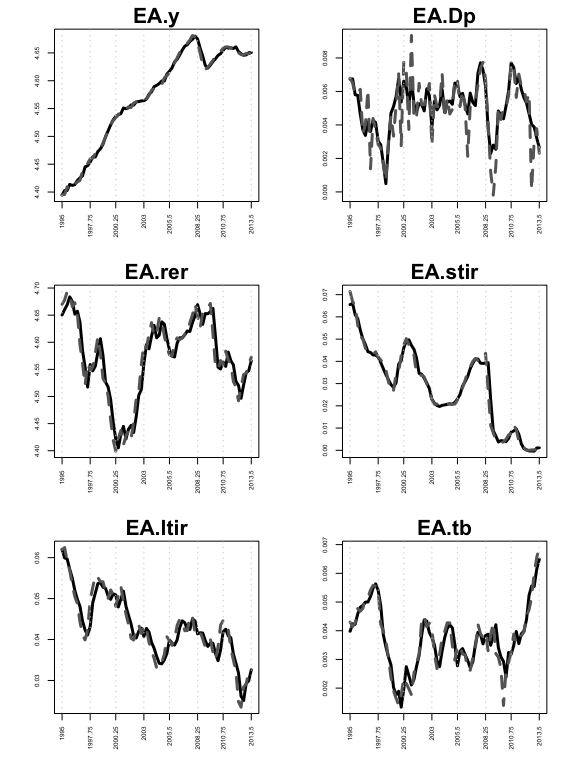In-sample fit for euro area variables We can estimate the model with two further priors on the unit models, the SSVS prior and the Minnesota prior. To give a concrete example, the SSVS prior can be invoked by typing: model.ssvs.1<-bgvar(Data=eerData, W=W.trade0012, draws=100, burnin=100, plag=1, prior="SSVS", hyperpara=NULL, SV=TRUE, thin=1, Ex=NULL, trend=TRUE, expert=list(save.shrink.store=TRUE), hold.out=0, eigen=1, verbose=TRUE ) One feature of the SSVS prior is that it allows us to look at the posterior inclusion probabilities to gauge the importance of particular variables. Per default, bgvar does not save the volatilities of the coefficients to save memory. If we set expert=list(save.shrink.store=TRUE) to TRUE (default is FALSE) then those probabilities are saved and posterior inclusion probabilities (PIPs) are computed. For example, we can have a look at the PIPs of the euro area model by typing: model.ssvs.1$cc.results$PIP$PIP.cc$EA ## y Dp rer stir ltir tb ## y_lag1 1.00 0.30 0.50 0.13 0.10 0.86 ## Dp_lag1 0.10 0.50 0.19 0.28 0.35 0.16 ## rer_lag1 0.30 0.78 1.00 1.00 0.08 0.55 ## stir_lag1 0.93 0.12 0.42 1.00 0.25 0.39 ## ltir_lag1 0.38 1.00 0.37 0.14 1.00 0.52 ## tb_lag1 0.14 0.56 0.79 0.09 0.16 1.00 ## y* 0.39 0.42 0.11 0.15 0.12 0.17 ## Dp* 0.33 0.44 0.10 0.33 0.12 0.34 ## rer* 0.19 0.27 1.00 0.06 0.09 0.22 ## stir* 0.19 0.48 0.26 0.05 0.13 0.07 ## ltir* 0.38 0.13 0.07 1.00 0.26 0.17 ## tb* 0.07 0.99 0.14 0.22 0.10 0.39 ## poil** 0.14 1.00 0.15 0.08 0.15 0.42 ## y*_lag1 0.41 0.21 0.16 0.07 0.11 0.16 ## Dp*_lag1 0.71 0.23 0.18 0.22 0.58 0.07 ## rer*_lag1 0.14 0.21 1.00 0.13 0.02 0.38 ## stir*_lag1 0.06 0.70 0.22 0.25 0.13 0.21 ## ltir*_lag1 1.00 1.00 0.24 1.00 0.34 0.15 ## tb*_lag1 0.13 0.79 1.00 0.59 0.03 0.81 ## poil**_lag1 0.56 0.77 0.17 0.06 0.11 0.41 ## cons 0.09 0.39 1.00 0.06 0.10 0.94 ## trend 0.10 1.00 0.12 0.06 0.14 0.79 The equations in the EA country model can be read column-wise with the rows representing the associated explanatory variables. The example shows that besides other variables, the trade balance (tb) is an important determinant of the real exchange rate (rer). We can also have a look at the average of the PIPs across all units: model.ssvs.1$cc.results$PIP$PIP.avg
##                     y        Dp       rer      stir  ltir        tb poil
## y_lag1      1.0000000 0.3066667 0.5866667 0.4600000 0.160 0.6300000 0.11
## Dp_lag1     0.2466667 0.5866667 0.1833333 0.4533333 0.315 0.1900000 0.10
## rer_lag1    0.2300000 0.6366667 1.0000000 0.4200000 0.225 0.5866667 0.71
## stir_lag1   0.7766667 0.1600000 0.4600000 1.0000000 0.435 0.2400000 0.15
## ltir_lag1   0.3050000 0.5500000 0.3800000 0.1900000 1.000 0.2850000 0.46
## tb_lag1     0.1300000 0.5633333 0.6033333 0.3766667 0.140 1.0000000 0.57
## y*          0.4966667 0.2400000 0.3866667 0.1866667 0.095 0.2800000 0.58
## Dp*         0.2333333 0.5366667 0.2866667 0.1833333 0.145 0.5233333 1.00
## rer*        0.3100000 0.2133333 0.6933333 0.1566667 0.390 0.1900000 0.39
## stir*       0.2200000 0.2933333 0.1933333 0.2900000 0.470 0.3133333 0.26
## ltir*       0.2066667 0.4166667 0.1133333 0.6133333 0.190 0.1333333 0.44
## tb*         0.1933333 0.6733333 0.3000000 0.2200000 0.130 0.3033333 0.84
## poil**      0.5200000 0.5350000 0.2350000 0.2500000 0.150 0.2600000  NaN
## y*_lag1     0.2233333 0.1166667 0.5266667 0.3733333 0.175 0.2133333 0.61
## Dp*_lag1    0.3000000 0.1266667 0.2333333 0.1266667 0.420 0.4066667 0.11
## rer*_lag1   0.3966667 0.1700000 0.5966667 0.1000000 0.365 0.2133333 0.19
## stir*_lag1  0.4066667 0.3266667 0.2000000 0.1766667 0.500 0.3333333 0.23
## ltir*_lag1  0.4433333 0.4666667 0.2000000 0.4233333 0.320 0.1000000 0.25
## tb*_lag1    0.1333333 0.5233333 0.5500000 0.3000000 0.150 0.3000000 0.14
## poil**_lag1 0.4750000 0.4700000 0.1600000 0.1550000 0.110 0.2200000  NaN
## cons        0.4966667 0.2700000 0.8033333 0.2400000 0.240 0.5066667 0.37
## trend       0.1300000 0.4766667 0.1766667 0.4566667 0.285 0.4433333 1.00
## poil_lag1   0.2900000 0.2200000 0.1200000 1.0000000 0.290 0.5400000 1.00

This shows that the same determinants for the real exchange rate appear as important regressors in other country models.

## Different Specifications of the Model

In this section we explore different specifications of the structure of the GVAR model. Other specification choices that relate more to the time series properties of the data, such as specifying different lags and priors are left for the reader to explore. We will use the SSVS prior and judge the different specifications by examining the posterior inclusion probabilities.

As a first modification, we could use different weights for different variable classes as proposed in Eickmeier and Ng (2015). For example we could use financial weights to construct weakly exogenous variables of financial factors and trade weights for real variables.

The eerData set provides us with a list of different weight matrices that are described in the help files.

Now we specify the sets of variables to be weighted:

variable.list<-list();variable.list$real<-c("y","Dp","tb");variable.list$fin<-c("stir","ltir","rer")

We can then re-estimate the model and hand over the variable.list via the argument expert:

# weights for first variable set tradeW.0012, for second finW0711
model.ssvs.2<-bgvar(Data=eerData,
plag=1,
draws=100,
burnin=100,
prior="SSVS",
SV=TRUE,
eigen=1,
expert=list(variable.list=variable.list,save.shrink.store=TRUE),
trend=TRUE
)

Another specification would be to include a foreign variable only when its domestic counterpart is missing. For example, when working with nominal bilateral exchange rates we probably do not want to include also its weighted average (which corresponds to something like an effective exchange rate). Using the previous model we could place an exclusion restriction on foreign long-term interest rates using Wex.restr which is again handed over via expert. The following includes foreign long-term rates only in those country models where no domestic long-term rates are available:

# does include ltir* only when ltir is missing domestically
model.ssvs.3<-bgvar(Data=eerData,
plag=1,
draws=100,
burnin=100,
prior="SSVS",
SV=TRUE,
eigen=1,
expert=list(Wex.restr="ltir",save.shrink.store=TRUE),
trend=TRUE,
)
 print(model.ssvs.3)
## ---------------------------------------------------------------------------
## Model Info:
## Prior: Stochastic Search Variable Selection prior (SSVS)
## Number of lags for endogenous variables: 1
## Number of lags for weakly exogenous variables: 1
## Number of posterior draws: 100/1=100
## Size of GVAR object: 0.5 Mb
## Trimming leads to 33 (33%) stable draws out of 100 total draws.
## ---------------------------------------------------------------------------
## Model specification:
##
## EA: y, Dp, rer, stir, ltir, tb, y*, Dp*, rer*, stir*, tb*, poil**, trend
## US: y, Dp, rer, stir, ltir, tb, poil, y*, Dp*, rer*, stir*, tb*, trend
## RU: y, Dp, rer, stir, tb, y*, Dp*, rer*, stir*, tb*, poil**, trend

Last, we could also use a different specification of oil prices in the model. Currently, the oil price is determined endogenously within the US model. Alternatively, one could set up an own standing oil price model with additional variables that feeds the oil price back into the other economies as exogenous variable (Mohaddes and Raissi 2019).

The model structure would then look something like in the Figure below: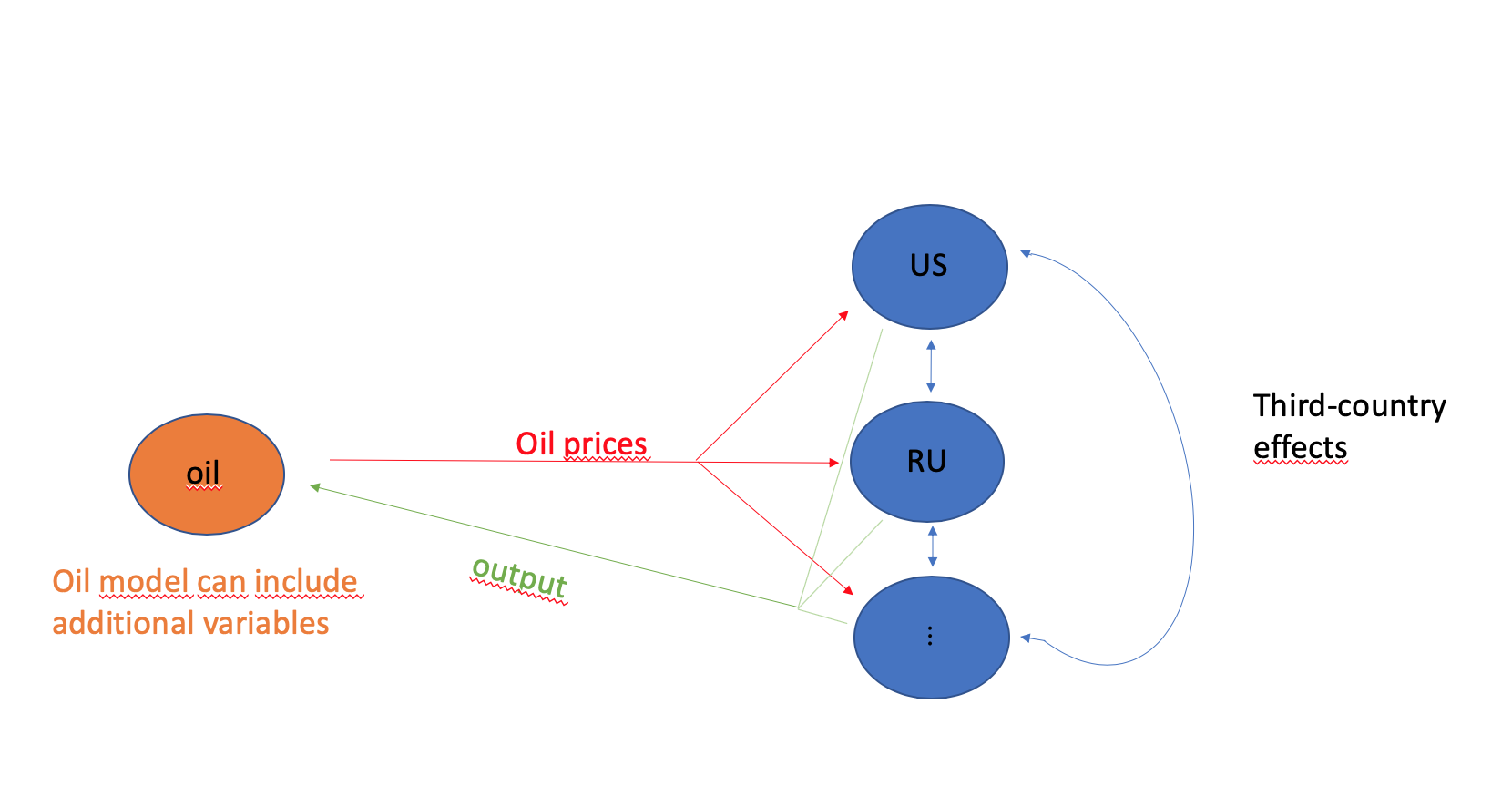“GVAR with oil prices modeled separately.”

For that purpose we have to remove oil prices from the US model and attach them to a separate slot in the data list. This slot has to have its own country label. We use ‘OC’ for “oil country”.

eerData2<-eerData
eerData2$OC<-eerData$US[,c("poil"),drop=FALSE] # move oil prices into own slot
eerData2$US<-eerData$US[,c("y","Dp", "rer" , "stir", "ltir","tb")] # exclude it from US m odel

Now we have to specify a list object that we label OC.weights. The list has to consist of three slots with the following names weights, variables and exo:

OC.weights<-list()
OC.weights$weights<-rep(1/3, 3) names(OC.weights$weights)<-names(eerData2)[1:3] # last one is OC model, hence only until 3
OC.weights$variables<-c(colnames(eerData2$OC),"y") # first entry, endog. variables, second entry weighted average of y from the other countries to proxy demand
OC.weights$exo<-"poil" The first slot, weights, should be a vector of weights that sum up to unity. In the example above, we simply use $$1/N$$, other weights could include purchasing power parities (PPP). The weights are used to aggregate specific variables that in turn enter the oil model as weakly exogenous. The second slot, variables, should specify the names of the endogenous and weakly exogenous variables that are used in the OC model. In the oil price example, we include the oil price (poil) as an endogenous variable (not contained in any other country model) and a weighted average using weights of output (y) to proxy world demand as weakly exogenous variable. Next, we specify via exo which one of the endogenous variables of the OC model are fed back into the other country models. In this example we specify poil. Last, we put all this information in a further list called OE.weights (other entity weights). This is done to allow for multiple other entity models (i.e., an oil price model, a joint monetary union model, etc.). It is important that the list entry has the same name as the other entity model, in our example OC. # other entities weights with same name as new oil country OE.weights <- list(OC=OC.weights) Now we can re-estimate the model where we pass on OE.weights via the expert argument. model.ssvs.4<-bgvar(Data=eerData2, W=W.trade0012, plag=1, draws=100, burnin=100, prior="SSVS", SV=TRUE, expert=list(OE.weights=OE.weights,save.shrink.store=TRUE), trend=TRUE ) and can compare the results of the four models by e.g., looking at the average PIPs. aux1<-model.ssvs.1$cc.results$PIP$PIP.avg;aux1<-aux1[-nrow(aux1),1:6]
aux2<-model.ssvs.2$cc.results$PIP$PIP.avg;aux2<-aux2[-nrow(aux2),1:6] aux3<-model.ssvs.3$cc.results$PIP$PIP.avg;aux3<-aux3[-nrow(aux3),1:6]
aux4<-model.ssvs.4$cc.results$PIP$PIP.avg;aux4<-aux4[-nrow(aux4),1:6] heatmap(aux1,Rowv=NA,Colv=NA, main="Model 1") heatmap(aux2,Rowv=NA,Colv=NA, main="Model 2") heatmap(aux3,Rowv=NA,Colv=NA, main="Model 3") heatmap(aux4,Rowv=NA,Colv=NA, main="Model 4")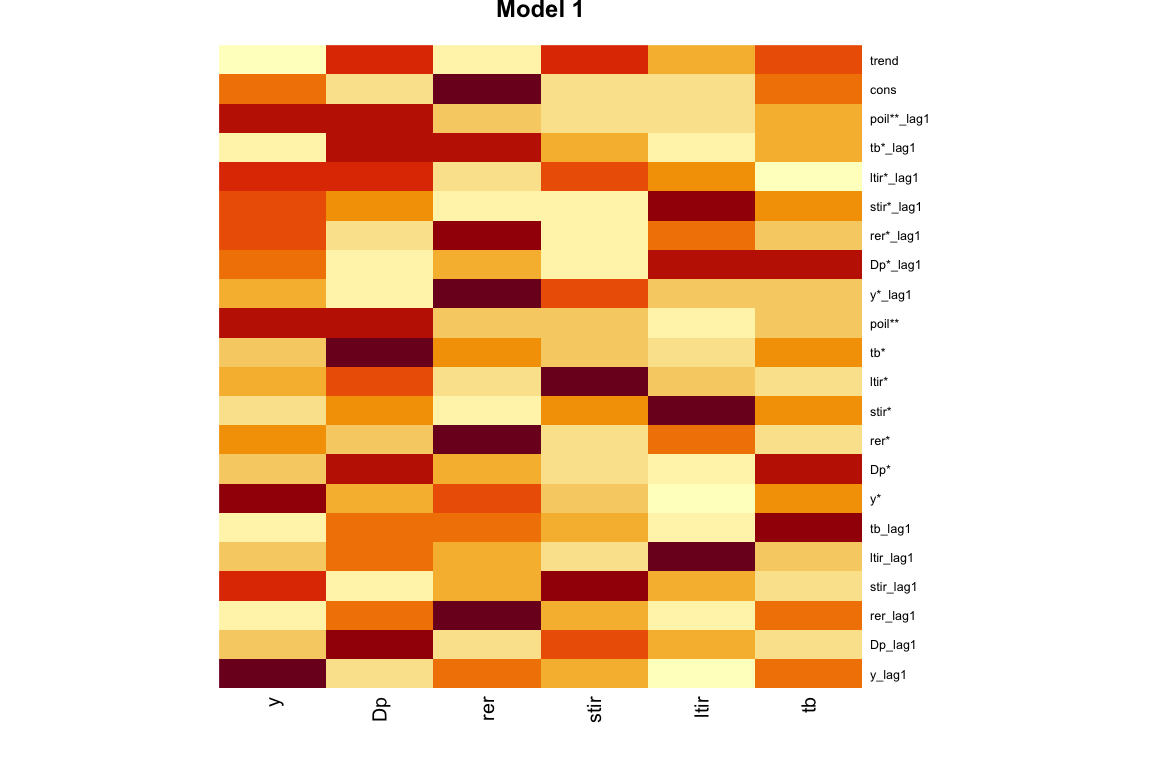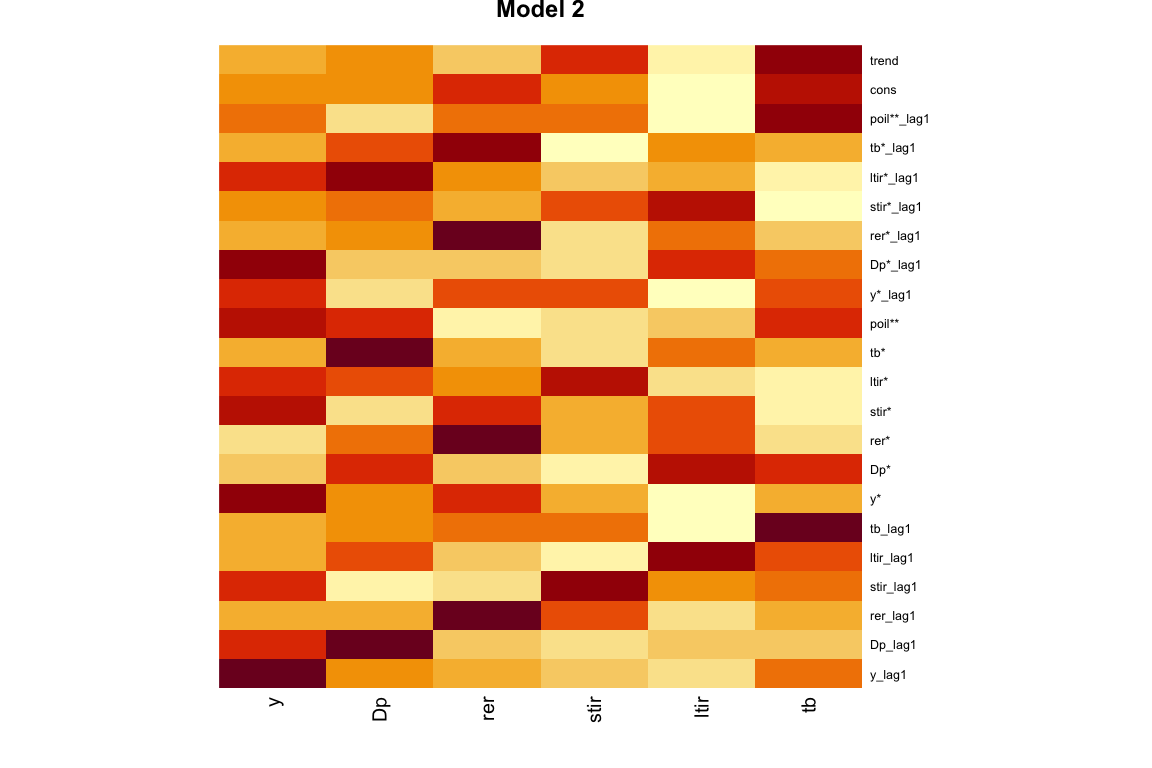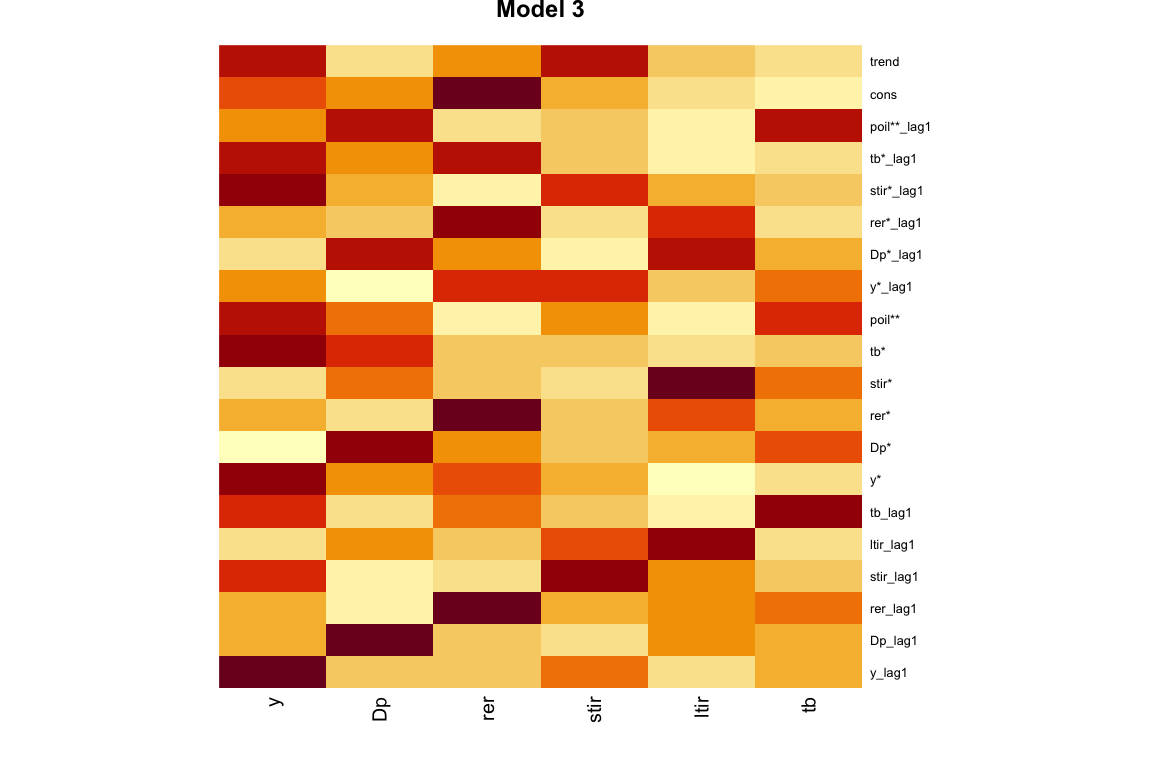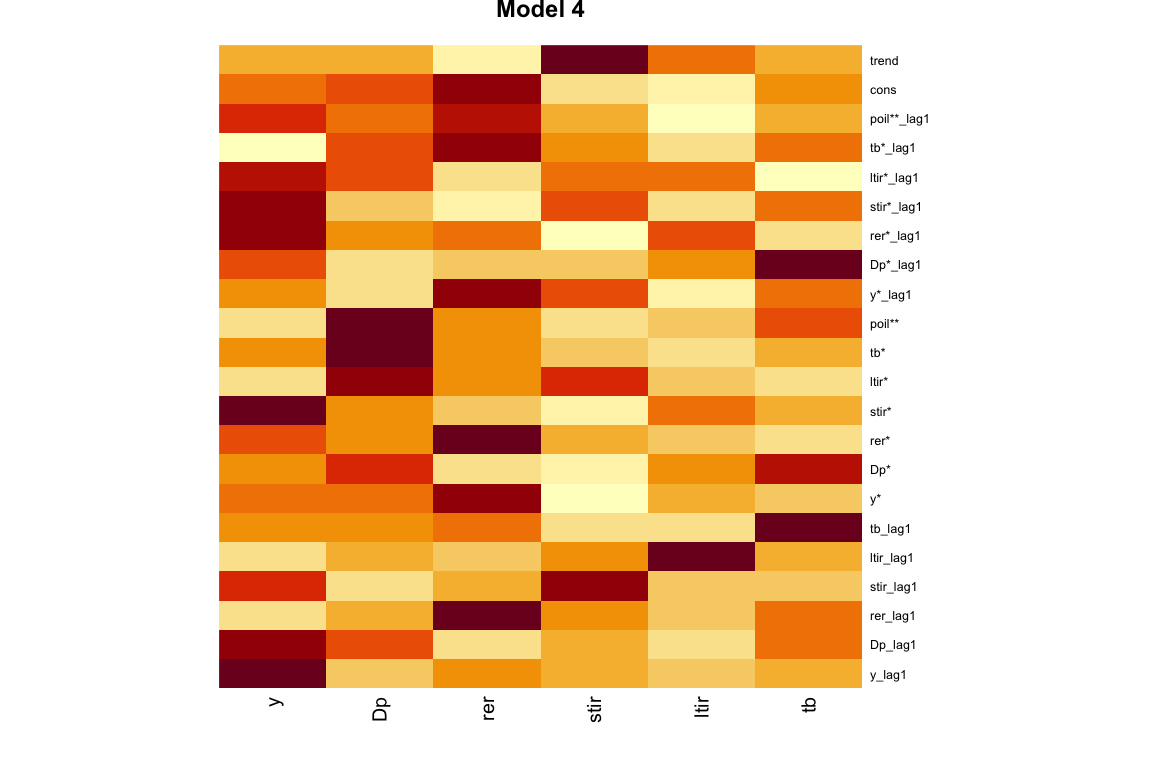Heatmaps of PIPs. We could also compare the models based on their fit, the likelihood, information criteria such as the DIC, residual properties or their forecasting performance. # Impulse response functions The package allows to calculate three different ways of dynamic responses, namely generalized impulse response functions (GIRFs) as in Pesaran and Shin (1998), orthogonalized impulse response functions using a Cholesky decomposition of the variance covariance matrix and finally impulse response functions given a set of user-specified sign restrictions. ## Recursive Identification and GIRFs Most of the GVAR applications deal with locally identified shocks. This implies that the shock of interest is orthogonal to the other shocks in the same unit model and hence can be interpreted in a structural way. There is still correlation between the shocks of the unit models, and these responses (the spillovers) are hence not fully structural (Eickmeier and Ng 2015). Hence some GVAR applications favor generalized impulse response functions, which per se do not rely on an orthogonalization. In BGVAR, responses to both types of shocks can be easily analyzed using the irf function. This function needs as input a model object (x), the impulse response horizon (n.ahead) and the default identification method is the recursive identification scheme via the Cholesky decomposition. Further arguments can be passed on using the wrapper expert and are discussed in the helpfiles. The following provides impulse response to all N shocks with unit scaling and using generalized impulse response functions: irf.chol<-irf(model.ssvs.1, n.ahead=24, expert=list(save.store=FALSE)) The results are stored in irf.chol$posterior, which is a four-dimensional array: $$K \times n.ahead \times nr.of shocks \times Q$$, with Q referring to the 50%, 68% and 95% quantiles of the posterior distribution of the impulse response functions. The posterior median of responses to the first shock could be accessed via irf.girf$posterior[,,1,"Q50"] Note that this example was for illustrational purposes; in most instances, we would be interested in a particular shock and calculating responses to all shocks in the system is rather inefficient. Hence, we can provide the irf function with more information. To be more precise, let us assume that we are interested in an expansionary monetary policy shock (i.e., a decrease in short-term interest rates) in the US country model. For that purpose, we can set up an shockinfo object, which contains information about which variable we want to shock (shock), the size of the shock (scale), the specific identification method(ident), and whether it is a shock applied in a single country or in multiple countries (global). We can use the helper function get_shockinfo() to set up a such a dummy object which we can subsequently modify according to our needs. The following lines of code are used for a negative 100 bp shock applied to US short term interest rates: # US monetary policy shock - Cholesky shockinfo_chol<-get_shockinfo("chol") shockinfo_chol$shock<-"US.stir"
shockinfo_chol$scale<--100 # US monetary policy shock - GIRF shockinfo_girf<-get_shockinfo("girf") shockinfo_girf$shock<-"US.stir"
shockinfo_girf$scale<--100 The shockinfo objects for Cholesky and GIRFs look exactly the same but have additionally an attribute which classifies the particular identification scheme. If we compare them, we notice that both have three columns defining the shock, the scale and whether it is defined as global shock. But we also see that the attributes differ which is important for the identification in the irf function. shockinfo_chol ## shock scale global ## 1 US.stir -100 FALSE shockinfo_girf ## shock scale global ## 1 US.stir -100 FALSE Now, we identify a monetary policy shock with recursive identification: irf.chol.us.mp<-irf(model.ssvs.1, n.ahead=24, shockinfo=shockinfo_chol, expert=list(save.store=TRUE)) The results are stored in irf.chol.us.mp. In order to save the complete set of draws, one can activate the save.store argument by setting it to TRUE within the expert settings (note: this may need a lot of storage). names(irf.chol.us.mp) ##  "posterior" "ident" "shockinfo" "rot.nr" "struc.obj" "model.obj" ##  "IRF_store" Again, irf.chol.us.mp$posterior is a $$K \times n.ahead \times nr.of shocks \times 7$$ object and the last slot contains the 50%, 68% and 95% credible intervals along with the posterior median. If save.store=TRUE, IRF_store contains the full set of impulse response draws and you can calculate additional quantiles of interest.

We can plot the complete responses of a particular country by typing:

plot(irf.chol.us.mp, resp="US", shock="US.stir")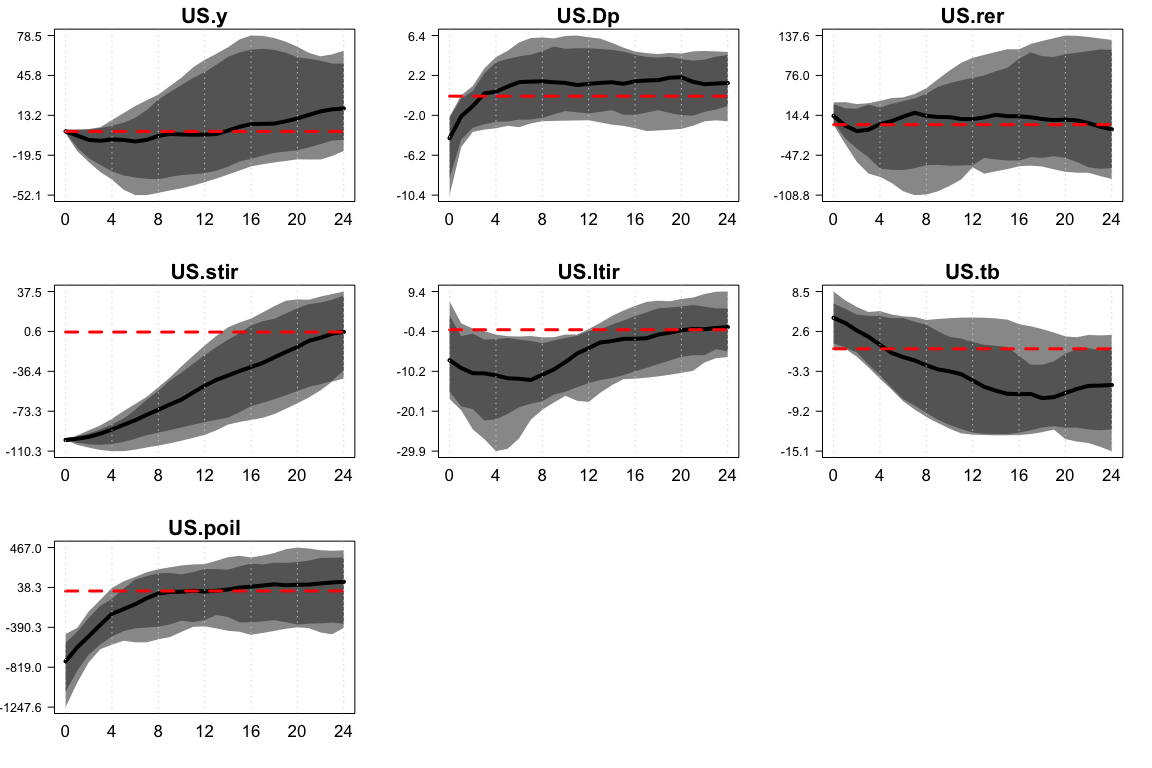Responses of US country model

The plot shows the posterior median response (solid, black line) along 50% (dark grey) and 68% (light grey) credible intervals.

We can also compare the Cholesky responses with GIRFs. For that purpose, let us look at a GDP shock.

# cholesky
shockinfo_chol       <- get_shockinfo("chol", nr_rows = 2)
shockinfo_chol$shock <- c("US.stir","US.y") shockinfo_chol$scale <- c(1,1)
# generalized impulse responses
shockinfo_girf       <- get_shockinfo("girf", nr_rows = 2)
shockinfo_girf$shock <- c("US.stir","US.y") shockinfo_girf$scale <- c(1,1)
# Recursive US GDP
irf.girf.us.y<-irf(model.ssvs.1, n.ahead=24, shockinfo=shockinfo_girf)
plot(irf.chol.us.y, resp="US.y", shock="US.y")
plot(irf.girf.us.y, resp="US.rer", shock="US.y")